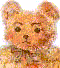BillHung.Net
 Lab 04 Circuit Extraction Files Part f VTC and Transcient Analysis * # FILE NAME: /HOME/AA/UGRAD/BILLHUNG/EE141/LAB04/INV1/HSPICES/EXTRACTED/ * NETLIST/INV1.C.RAW * NETLIST OUTPUT FOR HSPICES. * GENERATED ON FEB 23 13:40:45 2006 * FILE NAME: INVERTER_INV1_EXTRACTED.S. * SUBCIRCUIT FOR CELL: INV1. * GENERATED FOR: HSPICES. * GENERATED ON FEB 23 13:40:45 2006. * INCLUDE FILES .lib 'g25.mod' TT * params = vlow vhigh delay rise fall pulse_width period * example VIN IN GND PULSE 0 5 .5n .1n .1n .5n 2n VIN VIN 0 PULSE 0 2.5 5n 10p 10p 40n 80n VDD VDD! 0 2.5 M0 VOUT VIN VDD! VDD! PMOS L=240E-9 W=600E-9 AD=360.000007235128E-15 +AS=360.000007235128E-15 PD=1.7999999499807E-6 PS=1.7999999499807E-6 M=1 M1 VOUT VIN 0 0 NMOS L=240E-9 W=600E-9 AD=360.000007235128E-15 +AS=360.000007235128E-15 PD=1.7999999499807E-6 PS=1.7999999499807E-6 M=1 * ANALYSIS .tran 1ns 100ns .dc VIN 0 2.5 0.1 * END OF NETLIST .TEMP 25.0000 .OP .save .option nomod post *.OPTION INGOLD=2 ARTIST=2 PSF=2 *+ PROBE=0 .END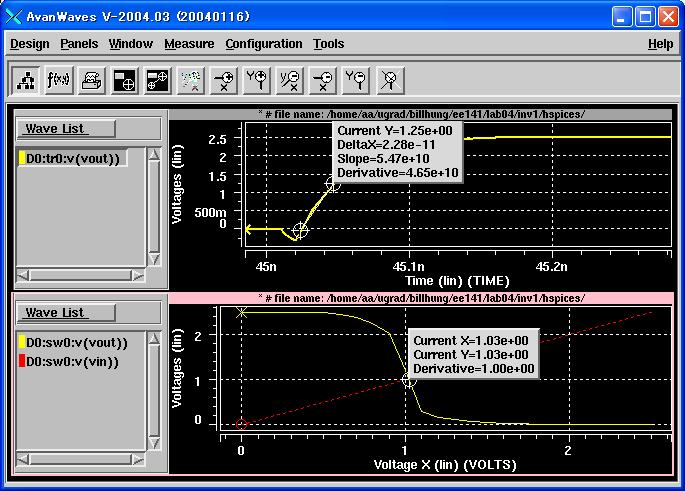hspiceFinal (extracted) * # FILE NAME: /HOME/AA/UGRAD/BILLHUNG/EE141/LAB04/INV1/HSPICES/EXTRACTED/ * NETLIST/INV1.C.RAW * NETLIST OUTPUT FOR HSPICES. * GENERATED ON FEB 23 13:40:45 2006 * FILE NAME: INVERTER_INV1_EXTRACTED.S. * SUBCIRCUIT FOR CELL: INV1. * GENERATED FOR: HSPICES. * GENERATED ON FEB 23 13:40:45 2006. M0 VOUT VIN VDD! VDD! TSMC25DP L=240E-9 W=600E-9 AD=360.000007235128E-15 +AS=360.000007235128E-15 PD=1.7999999499807E-6 PS=1.7999999499807E-6 M=1 M1 VOUT VIN 0 0 TSMC25DN L=240E-9 W=600E-9 AD=360.000007235128E-15 +AS=360.000007235128E-15 PD=1.7999999499807E-6 PS=1.7999999499807E-6 M=1 * INCLUDE FILES * END OF NETLIST .TEMP 25.0000 .OP .save .OPTION INGOLD=2 ARTIST=2 PSF=2 + PROBE=0 .END Hierarchical Schematics and Stimulation with Cadence In Lab5, the name is "bsl_example". the path is "~/ee141/lab4"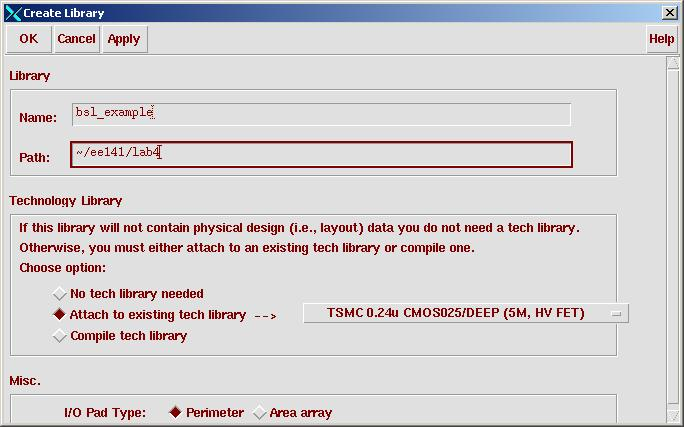new library "bsl_example"cell "mux21" view schematicget an instance of nmos4, trying to get the schematics like below. For pmos, type "pmos4" in Cell.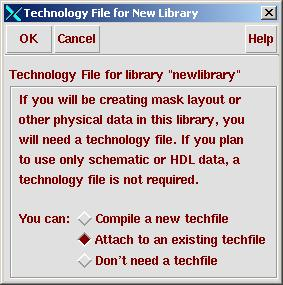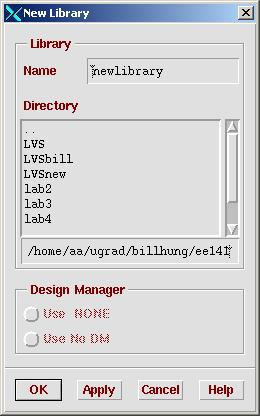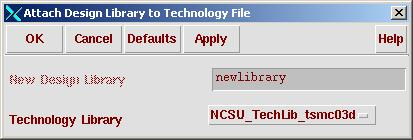Extract SPICE netlist from Cadence  a.       Open the inverter layout in Cadence and extract the layout by clicking Verify -> Extract… on the menu.  The extraction menu should come up and should look like the picture below. Make sure flat is selected from Extract Method. In the CIW there will be many commands, at the bottom there should “Total errors found: 0”. If you look in your Library Manager, there should be an additional view for your cell, extracted.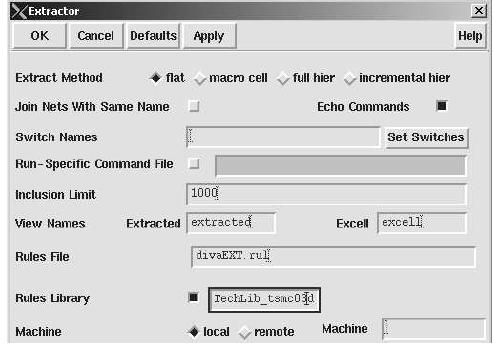b.       Examine the extracted inverter view. There will be simple outlines designating different layers in the layout. The blue polygons are the metal 1 wires, green outlines represent active area, red strips are poly, and orange boxes represent contacts. You should also see large red boxes and bold white lines that stand out from the outlines. These are the ports of your devices and the nets that connect them. c.       To netlist the extracted cell view, you will need to run Affirma Analog Environment by clicking on Tools -> Analog Environment. Analog Environment is a graphical interface for running and analyzing Spectre (a language similar to SPICE) simulations on circuits. You may find this tool useful for running analysis on your project. For now, we will use Analog Environment to generate SPICE netlists. In the Affirma Analog Circuit Design Environment window click Setup -> Environment…. In the window that opens select hspice from the Include/Stimulus File Syntax field and flat in the Netlist Type field, then click OK. Now go to Setup ->Simulator/Directory/Host…and change the Project Directory field to your lab directory then press OK. If you are prompted to save the state, go ahead press OK. Finally, click Simulation -> Netlist -> Create Final in Analog Environment. You will be able to see the netlist commands in the CIW and a “…successful” line then your netlist should open in a separate window. d.       Now go back to your command line and in your lab directory go to CELLNAME/hspiceS/extracted/netlist where CELLNAME is the name of your cell. In that directory, your extracted netlist will be called hspiceFinal. Examine the netlist and you will see all the MOS transistors. Make sure the NMOS and PMOS have the correct width, length, drain and source area, and perimeters. e.       We will not be using the NCSU model libraries, so comment out the lines:       .lib "/ncsu/cadence/local/models/hspice/public/publicModel/tsmc25dP" PMOS    .lib "/ncsu/cadence/local/models/hspice/public/publicModel/tsmc25dN" NMOS if they are present. Copy the EE141 spice model for TSMC 0.25 to the lab directory. > cp ~ee141/MODELS/g25.mod . Then add the line to reference the library, .lib ‘g25.mod’ TT for EE141 models. Change the NMOS and PMOS models from the “TSMC25DN” “TSMC25DP” to “NMOS” and “PMOS”, respectively. Finally comment out the Analog Artist options: .OPTION  INGOLD=2 ARTIST=2 PSF=2 +        PROBE=0   Hierarchical schematic entry in Composer http://bwrc.eecs.berkeley.edu/classes/icdesign/ee141_s06/CadenceLabs/hierarchy/hierarchy.htm "i", create nmos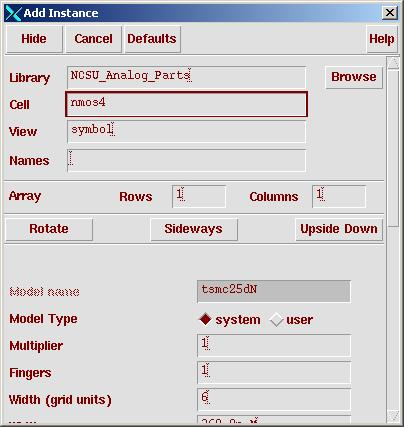"r" rotate, "w" wire, Add->Pin or "p" to put down pins. "l" to label wires in schematic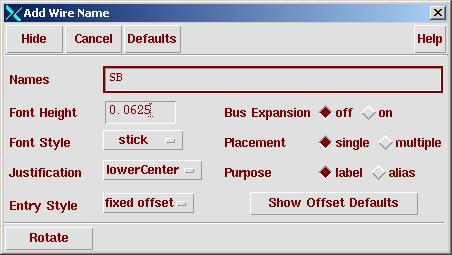Done drawing the schematic. Schematic check completed with no errors.Create Symbol View Design-Create Cellview->Form Cellview"OK"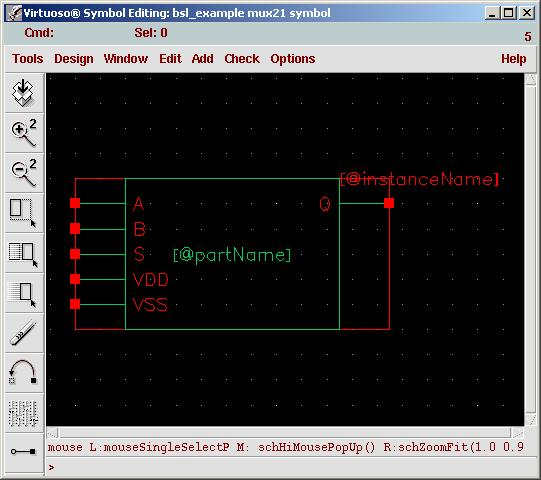Delete green box, redraw, move wires around.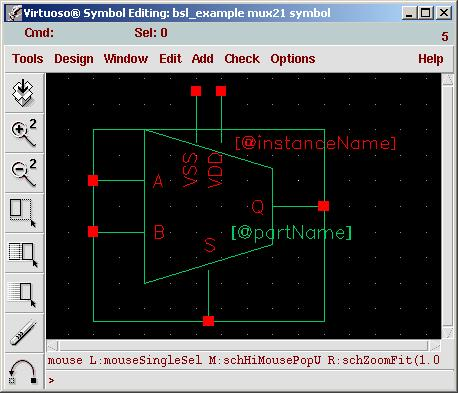Done. Create 4:1 mux In library manager. Cell=mux41, View=schematic.Instantiate three 2:1 mux cell.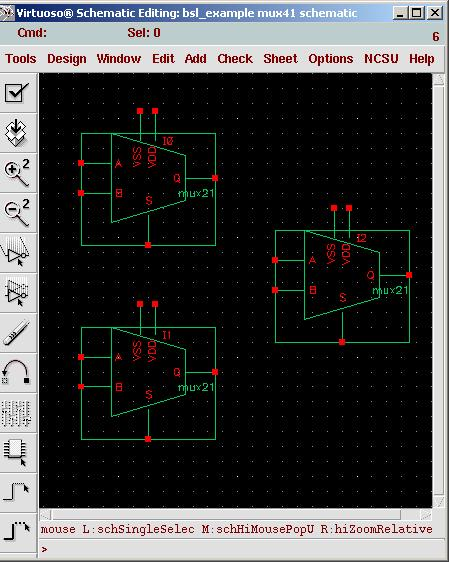Put pins, wires, and labels. "p" then D<3:0> for pin name. Output pin Q.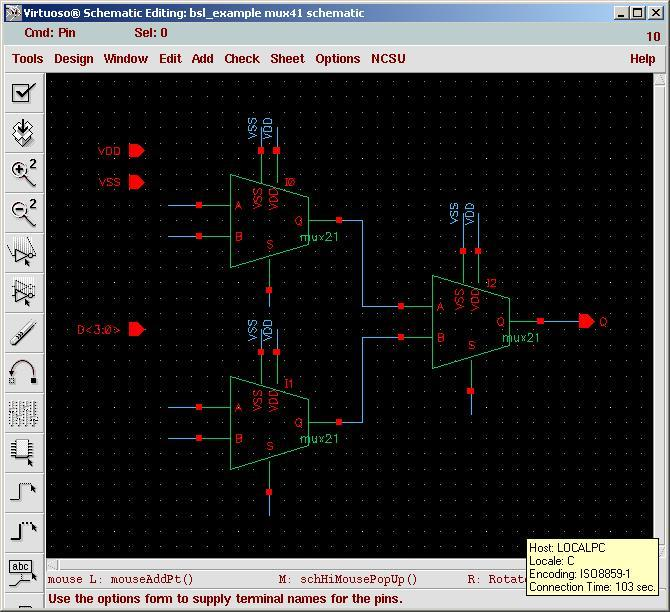Then wire the 4 input wires separately. "l" then name the wires <1><2><3><4>. Create S<0:1> for control bits, and "l" for S<0> and S<1>Done.  Schematic check completed with no errors. Make 4:1 mux symbole. Design-> Create Cellview->From Cellveiw. "OK"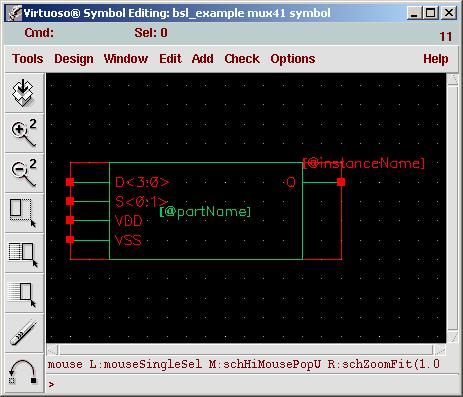Redraw the symbol.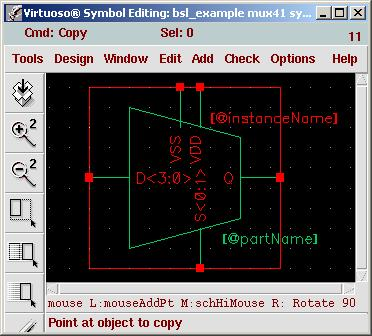Done.  Schematic check completed with no errors. Extrace Cint from hspice netlist Cint_inverter.sp Extract Cint for calculation of sizing parameter  .lib 'g25.mod' TT ***************************************** * Circuit * vdd * | * in---(Inverter)---out * |                  | *(VIN)               C1 * |                  | * 0                  0 ***************************************** * Parameter ***************************************** .param vddp=2.5 .param cload=100fF ***************************************** * Netlist ***************************************** * params = vlow vhigh delay rise fall pulse_width period * example VIN IN GND PULSE 0 5 .5n .1n .1n .5n 2n VIN in 0 PULSE 0 2.5 5n 10p 10p 40n 80n VDD vdd 0 'vddp' C1  out 0 'cload' .subckt INV VDD! GND VIN VOUT *M M0 VOUT VIN VDD! VDD!  PMOS  L=240E-9 W=600E-9 AD=360.000007235128E-15 +AS=360.000007235128E-15 PD=1.7999999499807E-6 PS=1.7999999499807E-6 M=1 M1 VOUT VIN GND GND  NMOS  L=240E-9 W=600E-9 AD=360.000007235128E-15 +AS=360.000007235128E-15 PD=1.7999999499807E-6 PS=1.7999999499807E-6 M=1 .ends Xinv1 vdd 0 in out INV   ***************************************** * Analysis ***************************************** *nomod= no model info from library .option nomod post=2 .meas tfall trig v(in) val='vddp/2' cross=1 targ v(in) val='vddp/2' cross=1 .tran 0.01ns 1ns sweep cload 0f 100f 1f     ***************************************** * END OF NETLIST ****************************************** .TEMP    25.0000    *.op makes hspice determines DC operating point .OP .save .ENDCint = 2.01e-10 / 3.80e3 - 5e-14 = 2.895 fF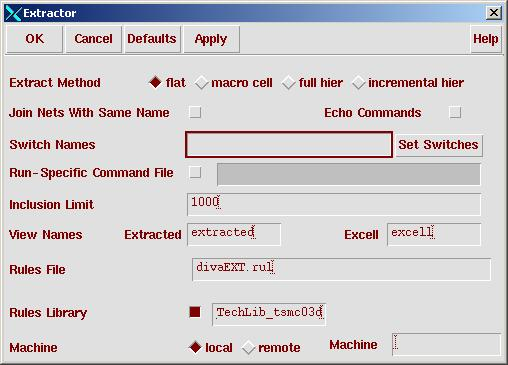Press Verify -> Extract Make sure flat is selected from Extract Method. Do Design Rule check (DRC). "OK" Total errors found: 0 The layout looks like this.To netlist the extracted cell view, you will need to run Affirma Analog Environment by clicking on Tools -> Analog Environment. Analog Environment is a graphical interface for running and analyzing Spectre (a language similar to SPICE) simulations on circuits. You may find this tool useful for running analysis on your project. For now, we will use Analog Environment to generate SPICE netlists. In the Affirma Analog Circuit Design Environment window click Setup -> Environment. In the window that opens select hspice from the Include/Stimulus File Syntax field and flat in the Netlist Type field, then click OK. Now go to Setup ->Simulator/Directory/Host and change the Project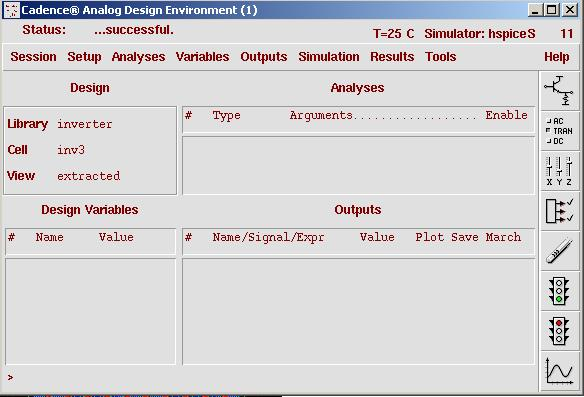...successful. Now go back to your command line and in your lab directory go to CELLNAME/hspiceS/extracted/netlist where CELLNAME is the name of your cell. In that directory, your extracted netlist will be called hspiceFinal. We will not be using the NCSU model libraries, so comment out the lines: .lib "/ncsu/cadence/local/models/hspice/public/publicModel/tsmc25dP" PMOS .lib "/ncsu/cadence/local/models/hspice/public/publicModel/tsmc25dN" NMOS if they are present. Copy the EE141 spice model for TSMC 0.25 to the lab directory. > cp ~ee141/MODELS/g25.mod . Then add the line to reference the library, .lib "g25.mod" TT for EE141 models. Change the NMOS and PMOS models from the ?TSMC25DN? ?TSMC25DP? to ?NMOS? and ?PMOS?, respectively. Finally comment out the Analog Artist options: .OPTION INGOLD=2 ARTIST=2 PSF=2 + PROBE=0  * # FILE NAME: /HOME/AA/UGRAD/BILLHUNG/CADENCE/SIMULATION/INV3/HSPICES/ * EXTRACTED/NETLIST/INV3.C.RAW * NETLIST OUTPUT FOR HSPICES. * GENERATED ON MAR 10 19:58:20 2006 M0 1 4 3 1 TSMC25DP L=240E-9 W=10.38E-6 AD=6.22799988664324E-12 +AS=3.73679993198595E-12 PD=11.580000318645E-6 PS=720.000002729648E-9 M=1 M1 3 4 1 1 TSMC25DP L=240E-9 W=10.38E-6 AD=3.73679993198595E-12 +AS=3.73679993198595E-12 PD=720.000002729648E-9 PS=720.000002729648E-9 M=1 M2 1 4 3 1 TSMC25DP L=240E-9 W=10.38E-6 AD=3.73679993198595E-12 +AS=3.73679993198595E-12 PD=720.000002729648E-9 PS=720.000002729648E-9 M=1 M3 3 4 1 1 TSMC25DP L=240E-9 W=10.38E-6 AD=3.73679993198595E-12 +AS=3.73679993198595E-12 PD=720.000002729648E-9 PS=720.000002729648E-9 M=1 M4 1 4 3 1 TSMC25DP L=240E-9 W=10.38E-6 AD=3.73679993198595E-12 +AS=3.73679993198595E-12 PD=720.000002729648E-9 PS=720.000002729648E-9 M=1 M5 3 4 1 1 TSMC25DP L=240E-9 W=10.38E-6 AD=3.73679993198595E-12 +AS=3.73679993198595E-12 PD=720.000002729648E-9 PS=720.000002729648E-9 M=1 M6 1 4 3 1 TSMC25DP L=240E-9 W=10.38E-6 AD=3.73679993198595E-12 +AS=3.73679993198595E-12 PD=720.000002729648E-9 PS=720.000002729648E-9 M=1 M7 3 4 1 1 TSMC25DP L=240E-9 W=10.38E-6 AD=3.73679993198595E-12 +AS=3.73679993198595E-12 PD=720.000002729648E-9 PS=720.000002729648E-9 M=1 M8 1 4 3 1 TSMC25DP L=240E-9 W=10.38E-6 AD=3.73679993198595E-12 +AS=3.73679993198595E-12 PD=720.000002729648E-9 PS=720.000002729648E-9 M=1 M9 3 4 1 1 TSMC25DP L=240E-9 W=10.38E-6 AD=3.73679993198595E-12 +AS=6.22799988664324E-12 PD=720.000002729648E-9 PS=11.580000318645E-6 M=1 M10 2 4 3 2 TSMC25DN L=240E-9 W=10.38E-6 AD=6.22799988664324E-12 +AS=3.73679993198595E-12 PD=11.580000318645E-6 PS=720.000002729648E-9 M=1 M11 3 4 2 2 TSMC25DN L=240E-9 W=10.38E-6 AD=3.73679993198595E-12 +AS=3.73679993198595E-12 PD=720.000002729648E-9 PS=720.000002729648E-9 M=1 M12 2 4 3 2 TSMC25DN L=240E-9 W=10.38E-6 AD=3.73679993198595E-12 +AS=3.73679993198595E-12 PD=720.000002729648E-9 PS=720.000002729648E-9 M=1 M13 3 4 2 2 TSMC25DN L=240E-9 W=10.38E-6 AD=3.73679993198595E-12 +AS=3.73679993198595E-12 PD=720.000002729648E-9 PS=720.000002729648E-9 M=1 M14 2 4 3 2 TSMC25DN L=240E-9 W=10.38E-6 AD=3.73679993198595E-12 +AS=3.73679993198595E-12 PD=720.000002729648E-9 PS=720.000002729648E-9 M=1 M15 3 4 2 2 TSMC25DN L=240E-9 W=10.38E-6 AD=3.73679993198595E-12 +AS=3.73679993198595E-12 PD=720.000002729648E-9 PS=720.000002729648E-9 M=1 M16 2 4 3 2 TSMC25DN L=240E-9 W=10.38E-6 AD=3.73679993198595E-12 +AS=3.73679993198595E-12 PD=720.000002729648E-9 PS=720.000002729648E-9 M=1 M17 3 4 2 2 TSMC25DN L=240E-9 W=10.38E-6 AD=3.73679993198595E-12 +AS=3.73679993198595E-12 PD=720.000002729648E-9 PS=720.000002729648E-9 M=1 M18 2 4 3 2 TSMC25DN L=240E-9 W=10.38E-6 AD=3.73679993198595E-12 +AS=3.73679993198595E-12 PD=720.000002729648E-9 PS=720.000002729648E-9 M=1 M19 3 4 2 2 TSMC25DN L=240E-9 W=10.38E-6 AD=3.73679993198595E-12 +AS=6.22799988664324E-12 PD=720.000002729648E-9 PS=11.580000318645E-6 M=1 * INCLUDE FILES * END OF NETLIST .TEMP 25.0000 .OP .save .OPTION INGOLD=2 ARTIST=2 PSF=2 + PROBE=0 .END The Edited Resizing HSPICE Netlist * # FILE NAME: /HOME/AA/UGRAD/BILLHUNG/CADENCE/SIMULATION/INV3/HSPICES/ * EXTRACTED/NETLIST/INV3.C.RAW * NETLIST OUTPUT FOR HSPICES. * GENERATED ON MAR 10 19:58:20 2006 M0 1 4 3 1 PMOS L=240E-9 W=10.38E-6 AD=6.22799988664324E-12 +AS=3.73679993198595E-12 PD=11.580000318645E-6 PS=720.000002729648E-9 M=1 M1 3 4 1 1 PMOS L=240E-9 W=10.38E-6 AD=3.73679993198595E-12 +AS=3.73679993198595E-12 PD=720.000002729648E-9 PS=720.000002729648E-9 M=1 M2 1 4 3 1 PMOS L=240E-9 W=10.38E-6 AD=3.73679993198595E-12 +AS=3.73679993198595E-12 PD=720.000002729648E-9 PS=720.000002729648E-9 M=1 M3 3 4 1 1 PMOS L=240E-9 W=10.38E-6 AD=3.73679993198595E-12 +AS=3.73679993198595E-12 PD=720.000002729648E-9 PS=720.000002729648E-9 M=1 M4 1 4 3 1 PMOS L=240E-9 W=10.38E-6 AD=3.73679993198595E-12 +AS=3.73679993198595E-12 PD=720.000002729648E-9 PS=720.000002729648E-9 M=1 M5 3 4 1 1 PMOS L=240E-9 W=10.38E-6 AD=3.73679993198595E-12 +AS=3.73679993198595E-12 PD=720.000002729648E-9 PS=720.000002729648E-9 M=1 M6 1 4 3 1 PMOS L=240E-9 W=10.38E-6 AD=3.73679993198595E-12 +AS=3.73679993198595E-12 PD=720.000002729648E-9 PS=720.000002729648E-9 M=1 M7 3 4 1 1 PMOS L=240E-9 W=10.38E-6 AD=3.73679993198595E-12 +AS=3.73679993198595E-12 PD=720.000002729648E-9 PS=720.000002729648E-9 M=1 M8 1 4 3 1 PMOS L=240E-9 W=10.38E-6 AD=3.73679993198595E-12 +AS=3.73679993198595E-12 PD=720.000002729648E-9 PS=720.000002729648E-9 M=1 M9 3 4 1 1 PMOS L=240E-9 W=10.38E-6 AD=3.73679993198595E-12 +AS=6.22799988664324E-12 PD=720.000002729648E-9 PS=11.580000318645E-6 M=1 M10 2 4 3 2 NMOS L=240E-9 W=10.38E-6 AD=6.22799988664324E-12 +AS=3.73679993198595E-12 PD=11.580000318645E-6 PS=720.000002729648E-9 M=1 M11 3 4 2 2 NMOS L=240E-9 W=10.38E-6 AD=3.73679993198595E-12 +AS=3.73679993198595E-12 PD=720.000002729648E-9 PS=720.000002729648E-9 M=1 M12 2 4 3 2 NMOS L=240E-9 W=10.38E-6 AD=3.73679993198595E-12 +AS=3.73679993198595E-12 PD=720.000002729648E-9 PS=720.000002729648E-9 M=1 M13 3 4 2 2 NMOS L=240E-9 W=10.38E-6 AD=3.73679993198595E-12 +AS=3.73679993198595E-12 PD=720.000002729648E-9 PS=720.000002729648E-9 M=1 M14 2 4 3 2 NMOS L=240E-9 W=10.38E-6 AD=3.73679993198595E-12 +AS=3.73679993198595E-12 PD=720.000002729648E-9 PS=720.000002729648E-9 M=1 M15 3 4 2 2 NMOS L=240E-9 W=10.38E-6 AD=3.73679993198595E-12 +AS=3.73679993198595E-12 PD=720.000002729648E-9 PS=720.000002729648E-9 M=1 M16 2 4 3 2 NMOS L=240E-9 W=10.38E-6 AD=3.73679993198595E-12 +AS=3.73679993198595E-12 PD=720.000002729648E-9 PS=720.000002729648E-9 M=1 M17 3 4 2 2 NMOS L=240E-9 W=10.38E-6 AD=3.73679993198595E-12 +AS=3.73679993198595E-12 PD=720.000002729648E-9 PS=720.000002729648E-9 M=1 M18 2 4 3 2 NMOS L=240E-9 W=10.38E-6 AD=3.73679993198595E-12 +AS=3.73679993198595E-12 PD=720.000002729648E-9 PS=720.000002729648E-9 M=1 M19 3 4 2 2 NMOS L=240E-9 W=10.38E-6 AD=3.73679993198595E-12 +AS=6.22799988664324E-12 PD=720.000002729648E-9 PS=11.580000318645E-6 M=1 * INCLUDE FILES .lib '/home/ff/ee141/MODELS/g25.mod' TT * params = vlow vhigh delay rise fall pulse_width period * example VIN IN GND PULSE 0 5 .5n .1n .1n .5n 2n VIN 4 0 PULSE 0 2.5 5n 10p 10p 40n 80n VDD 1 0 2.5 VGND 2 0 0 cload 3 0 50fF * ANALYSIS .tran 1ns 100ns * END OF NETLIST .TEMP 25.0000 .OP .save .option nomod post=2 *.OPTION INGOLD=2 ARTIST=2 PSF=2 *+ PROBE=0 .END